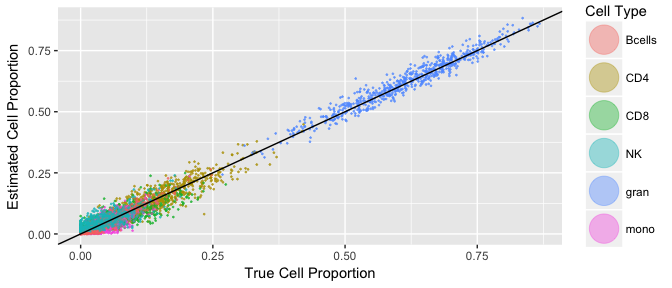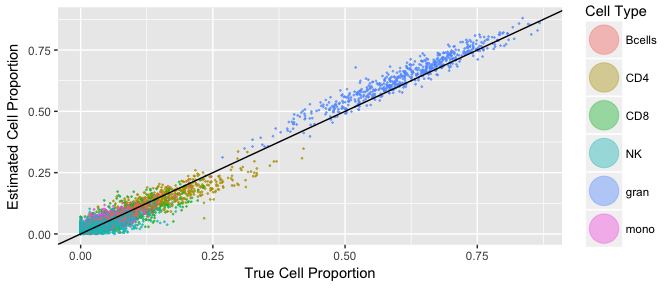BiSect: Infering Cell Type Compositon

2018-04-15

When conducting Epigenome Wide Association Studies (EWAS) on methylation data, it is important to account for the cell type heterogeneity in the samples, as failing to do so can result in biases and false positives. A common and simple way to do so, is the inclusion of the cell type composition of each sample as covariate in the linear model used for the EWAS. BiSect is an accurate method for inferring the cell compositon of samples from their methylation data. It is specifically taylored to work on methylation sequencing data, and therefore provides calibrated estimates even in low-coverage setting. This package implements two modes, a supervised mode, for estimating the cell composition using a reference that contains the probability for methylation in each isolated cell type (a reference for blood samples is provided), and an unsupervised mode, that estimates the reference, but requires the cell composition for a subset of the samples.

Supervised Mode: Using a Reference

Using the supervised mode is pretty straight forward. First, we need two matrices: one with the number of methylated reads, and one with the number of total reads, for each sample and each site. This example was subsampled from array data provided in Hannum et al. (2013). The rows are samples and the columns are CpG sites:

library(bisect)

methylation <- as.matrix(methylation_GSE40279)

dim(methylation)
#>  650 241
#>  650 241

#>     [,1] [,2] [,3] [,4] [,5]
#> X1     1   59   37  105    4
#> X2    67   18   15    0   44
#> X3    40   18  105   62   31
#> X4    14   33   17   59   19
#> X5    10    0    4    2   75
#> X6    13   11  112   65    0
#> X7     4   18    5    1   20
#> X8    27   49   63  145    1
#> X9    24   53   19   20   79
#> X10    3  105   50   56   57

We also need a reference with the proabability for methylation in each site, in each pure cell type. Here we used the reference of D. C. Koestler et al. (2016).

dim(reference_blood)
#>  241   7
reference_blood[1:10, ]
#>            ID    CD4.    CD8.     mono   Bcells       NK       gran
#> 1  cg00084577 0.86282 0.83790 0.741340 0.838560 0.848500 0.20808080
#> 2  cg00162673 0.85794 0.83649 0.599850 0.016539 0.737560 0.83139797
#> 3  cg00183468 0.82542 0.80833 0.777650 0.122550 0.695830 0.65098946
#> 4  cg00219921 0.84441 0.21809 0.908130 0.926950 0.761270 0.90566373
#> 5  cg00265360 0.25794 0.11075 0.097137 0.117310 0.098614 0.11137636
#> 6  cg00324520 0.76324 0.59521 0.876540 0.734150 0.613840 0.66442231
#> 7  cg00328720 0.85807 0.89810 0.426440 0.891920 0.830620 0.86784993
#> 8  cg00460983 0.81657 0.86633 0.152830 0.757450 0.752670 0.60345089
#> 9  cg00576774 0.12268 0.24344 0.036578 0.087278 0.144650 0.04545844
#> 10 cg00661777 0.31831 0.14463 0.090613 0.052831 0.063331 0.72754751

Pi <- as.matrix(reference_blood[,-1]) # For running Bisect we don't need the cg IDs, and we need the reference as a matrix.

Notice that we have one the sites in the three matrices (methylation, total_reads and the reference) need to appear in the same order.

The last optional thing is the hyper-parameters for the prior dirichlet distribution imposed on the cell types proportions. We provide recommended values for blood samples and the above cell types that were estimated by fitting a dirichlet distribution to cell counts data.

print(alpha_blood)
#>   2.5392  1.7934  0.7240  0.7404  1.8439 15.0727

Now we are ready to run bisect:

results <- bisect_supervised(methylation, total_reads, Pi, alpha_blood, iterations = 200)
#> ===========================================================================

#>            [,1]       [,2]        [,3]         [,4]       [,5]      [,6]
#> [1,] 0.07014205 0.01042750 0.039847588 8.373833e-04 0.01911895 0.8596265
#> [2,] 0.12032723 0.06287661 0.015075481 1.414233e-01 0.04433674 0.6159607
#> [3,] 0.19795639 0.04119062 0.091364136 1.128116e-01 0.05120256 0.5054747
#> [4,] 0.07779847 0.04038553 0.082421768 6.925135e-05 0.07119539 0.7281296
#> [5,] 0.11314241 0.13117025 0.028206084 4.652406e-04 0.04872687 0.6782892
#> [6,] 0.06582556 0.03990614 0.007337862 4.939628e-04 0.03666059 0.8497759

Before subsampling the dataset of Hahnum el al. we used the method by Houseman et al. (2012) to estimate the cell composition from the array data. Because array data contains many thausands of probes at each site, the estimate is fairly accurate. Now we can compare the results of BiSect to a baseline:

library(dplyr)
#>
#> Attaching package: 'dplyr'
#> The following objects are masked from 'package:stats':
#>
#>     filter, lag
#> The following objects are masked from 'package:base':
#>
#>     intersect, setdiff, setequal, union
library(ggplot2)
library(tidyr)

# organizing the results to a data.frame that works with ggplot2
get_visualization_dataframe <- function(bisect_results, true_cell_counts) {
estimates_bin <- as.data.frame(bisect_results)
true_cell_counts <- as.data.frame(true_cell_counts)

colnames(estimates_bin) <- c("CD4", "CD8", "mono", "Bcells", "NK", "gran")
colnames(true_cell_counts) <- c("CD4", "CD8", "mono", "Bcells", "NK", "gran")

gathered_estimates_bin <- estimates_bin %>% gather("CD4", "CD8", "mono", "Bcells", "NK", "gran", key = "cell_type", value = "estimate_norm")
gathered_truth <- true_cell_counts %>% gather("CD4", "CD8", "mono", "Bcells", "NK", "gran", key = "cell_type", value = "truth")

gathered_estimates_bin <- gathered_estimates_bin %>% mutate(method = "bin")
colnames(gathered_estimates_bin) <- c("cell_type", "estimate", "method")

estimates <- rbind(gathered_estimates_bin)
truth <- rbind(gathered_truth, gathered_truth)

results <- cbind(truth, select(estimates, "estimate", "method"))

return(results)
}

visualization_result <- get_visualization_dataframe(results, baseline_GSE40279)

# plot a scatter plot of true cell types vs estimated.  Looks pretty good!
visualization_result %>% ggplot(aes(truth, estimate, color=cell_type)) + geom_point(size=0.2, alpha = 0.4) +
geom_abline(intercept = 0, slope = 1) + xlab("True Cell Proportion") + ylab("Estimated Cell Proportion") +
guides(colour = guide_legend(override.aes = list(size=10))) + scale_color_discrete(name = "Cell Type")Semi-Supervised Mode: Using a Sub-Sample With Known Cell Composition

Using the semi-supervised mode is pretty straight-forward as well, only this time we need 5 matrices: the methylated and total reads for the samples with unknown cell composition, the same two matrices for the samples with known cell composition, and the matrix of cell composition, for the samples for whom it is known.

For the purpose of this tutorial we will simply use a randomly selected subset of the samples in GSE40279 to use as known samples. First, we choose the random samples:

set.seed(4321)

# Choose 50 random individuals with known cell type composition
n_known_samples <- 50
known_samples_indices <- sample.int(nrow(baseline_GSE40279), size = n_known_samples)
known_samples <- as.matrix(baseline_GSE40279[known_samples_indices, ])

Now we can fit a Dirichlet distribution to them, to be used as a prior for the rest of the samples:

# Fit a dirichlet distirbutio nto the known samples to use as a prior
fit_dirichlet <- sirt::dirichlet.mle(as.matrix(known_samples))
alpha <- fit_dirichlet$alpha And all that is left is to seperate the methylated and total reads matrices and run bisect: # Organize all the matrices such that the known samples are the first 50 rows. methylation_known <- methylation_GSE40279[known_samples_indices, ] methylation_unknown <-methylation_GSE40279[-known_samples_indices, ] total_known <- total_reads_GSE40279[known_samples_indices, ] total_unknown <- total_reads_GSE40279[-known_samples_indices, ] # Run Bisect, making sure to supply the number of known individuals. results <- bisect_semi_suprevised(methylation_unknown, total_unknown, methylation_known, total_known, known_samples, alpha, iterations = 200) #>  "estimating reference ........." #> =========================================================================== "estimating cell composition ........." #> =========================================================================== This time results is a list containing both the cell type estimates for the unknown samples, and the estimated reference. Let us plot the estimated cell types against our full baseline: library(ggplot2) visualization_result <- get_visualization_dataframe(results$P, baseline_GSE40279[-known_samples_indices,])

# plot a scatter plot of true cell types vs estimated.  Looks pretty good!
visualization_result %>% ggplot(aes(truth, estimate, color=cell_type)) + geom_point(size=0.2, alpha = 0.4) +
geom_abline(intercept = 0, slope = 1) + xlab("True Cell Proportion") + ylab("Estimated Cell Proportion") +
guides(colour = guide_legend(override.aes = list(size=10))) + scale_color_discrete(name = "Cell Type")And we can also take a look at our estimate for the reference:

estimated_reference <- results\$Pi